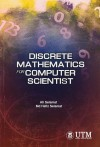# Discrete Mathematics for Computer Scientist by Ali Selamat, Md Hafiz Selamat(price excluding 0% GST)
Category:
ISBN: 978-983-52-1256-7
Publisher:
File Size: 10.06 MB
Format: EPUB (e-book)
DRM: Applied (Requires eSentral Reader App)
(price excluding 0% GST)

### Synopsis

This book serves as an introduction to computer sciences students in the undergraduate levels. It will be used as the foundation to understand the discrete mathematic in developing the logic of computer programs. Since there are also similar undergraduate computer science programmes in other local and overseas institutions, this book is expected to find wider local and international readership. Topics covered in this book include set theory and relations, functions sequence and string, propositional logic, predicate logic, matrices, graph theory and trees. As the book serves as an introductory level to computer science students, it is expected that once the students are already familiar with the presented contents, it will enable them to understand the advanced topics in computer science such as advanced theory of computer science and computational complexity theories. The chapters in this book have been organized for the students to learn and understand the main concepts of discrete mathematics for developing computer applications during the period of their studies. In information technology (IT) and computer science fields, most of information is represented in digital electronics based on the basic knowledge of discrete mathematics. Therefore, discrete mathematics is one of the relevant courses to support students for better learning and understanding the nature of computer science and IT. It is expected that by using the materials presented in this book students should be able to write statements using mathematical language, develop mathematical arguments using logic, apply the concept of integers and its role in modeling and solving problems in IT, and apply the concept of graph and tree for modeling and solving problems related to real situations.